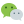# 最大公约数(Greatest Common Divisor)

## 最大质因数法

``````function getGcd(a, b) {
for (let i = a; i > 0; i--) {
for (let j = b; j > 0; j--) {
if (a % i === 0 && b % j === 0 && i === j) {
return j;
}
}
}
}
``````

## 辗转相除法

``````function getGcd(a, b) {
let max = Math.max(a, b);
let min = Math.min(a, b);
if (max % min === 0) {
return min;
} else {
return getGcd(max % min, min);
}
}
``````

# 求最小公倍数

``````function getLcm(a, b) {
return a * b / getGcd(a, b);
}
``````

### 评论：微信公众号：程序骑士